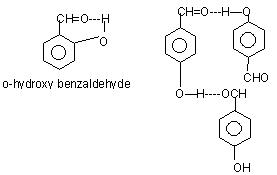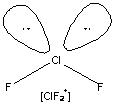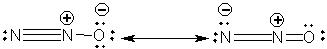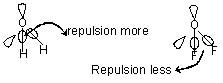Click to Chat

1800-1023-196

+91-120-4616500

CART 0

• 0

MY CART (5)

Use Coupon: CART20 and get 20% off on all online Study Material

ITEM
DETAILS
MRP
DISCOUNT
FINAL PRICE
Total Price: Rs.

There are no items in this cart.
Continue Shopping```Solved Problems of Chemical BondingProb 1. Arrange the bonds in order of increasing ionic character in the molecules:

LiF, K2O, N2, SO2 and ClF3

Solution: N2 < ClF3 < SO2 < K2O < LiF

Prob 2. Arrange the following in order of increasing ionic character:

C-H, F-H, Br-H, Na-I, K-F and Li-Cl

Solution: C-H < Br-H < F-H < Na-I < Li-Cl < K-F

Prob 3. Predict the shapes of the following ions

(a) BeF3-                  (b) BF4-

(c) IF4-                     (d) IBr2-

Solution:

(a) Triangular

(b) Tetrahedral

(c) Square planar

(d) Linear

Prob 4. Arrange the following in increasing order of stability O2, O2+, O2-, O22-

Solution: O22- < O2- < O2 < O2+

Calculate first the bond order which is as follows

O2 → 2, O2+ → 2.5, O2- → 1.5, O22- → 1 & then arrange according to increasing bond order.

Prob 5. Arrange the following:

(i) N2, O2, F2, Cl2 in increasing order of bond dissociation energy.

(ii) Increasing strength of hydrogen bonding (X – H – X):

O, S, F, Cl, N

Solution: (i) F2 < Cl2 < O2 < N2 (ii) Cl < S < N < O < F

Prob 6. Explain the following o - hydroxy benzaldehyde is liquid at room temperature while p - hydroxy benzaldehyde is high melting solid.

Solution: There is intramolecular H bonding in o - hydroxy benzaldehyde while intermolecular hydrogen bonding in p-hydroxy benzaldehydeProb 7. Explain why ClF2- is linear but ClF2+ is a bent molecular ion?

Solution: Chlorine atom lies in sp3d hybrid state. Three lone pairs are oriented along the corners of triangular planeChlorine atom lies in sp3 hybrid state. Two lone pairs are oriented along two corners of tetrahedralProb 8. AlF3 is ionic while AlCl3 is covalent.

Solution: Since F– is smaller in size, its polarisability is less and therefore it is having more ionic character. Whereas Cl being large in size is having more polarisability and hence more covalent character.

Prob 9. Write down the resonance structure of nitrous oxide

Solution:Prob 10. Explain why BeH2 molecule has zero dipole moment although the Be-H bonds are    polar.

Solution: BeH2 is a linear molecule (H-Be-H) with bond angle equal to 180o. Although the Be-H bonds are polar on account of electronegativity difference between Be and H atoms, the bond polarities cancel with each other. The molecule has resultant dipole moment of zero.

Prob 11. Why the bond angle of H – C – H in methane (CH4) is 109° 28’ while H – N – H bond angle in NH3 is 107° though both carbon and nitrogen are sp3 hybridized

Solution: In CH4 there are 4 bond pair of electrons while in NH3 are 3 bond pair of electrons and 1 lone pair of electrons. Since bond pair bond pair repulsion is less than lone pair bond pair repulsion, in NH3 bond angle is reduced from 109°28’ to 107°.

Prob 12. Why bond angle in NH3 is 107° while in H2O it is 104.5°?

Solution: In NH3, central nitrogen atom bears only one lone pair of electrons whereas in H2O central oxygen atom bears two lone pair of electrons.

Since the repulsion between lone pair-lone pair and lone pair – bond pair is more than that between bond pair-bond pair, the repulsion in H2O is much greater than that in NH3 which results in contraction of bond angle from 109°28” to 104.5° in water while in NH3 contraction is less i.e. from 109°28” to 107°.

“If the electronegativity of the peripheral atoms is more, then the bond angle will be less”.
For example if we consider NH3 and NF3, F – N – F bond angle will be lower than H – N – H bond angle. This is because in NF3 the bond pair is displaced more towards F and in NH3 it is displaced more towards N. So accordingly the b.p. – b.p. interaction is less in NF3 and more in NH3.

Prob 13. The bond angle of H2O is 104° while that that of F2O is 102°.

Solution:Both H2O and F2O have a lone pair of electrons. But fluorine being highly electronegative, the bond pair of electrons are drawn more towards F in F2O, whereas in H2O it is drawn towards O. So in F2O the bond pairs being displaced away from the central atom, has very little tendency to open up the angle. But in H2O this opening up is more as the bond pair electrons are closer to each other. So bond Ð of F2O is less than H2O.Prob 14.  Predict the hybridization for the central atom in POCl3, OSF4, OIF5.

Solution:

Total No. of V.E. = 5+6+21/8 = 32/8 = 4

So, hybridization = sp3

OSF4 = 6+6+28/8 = 40/8 = 5

So, hybridization of s = dsp3

OIF5 = 6+7+35/8 = 48/8 = 6

So, hybridization of I = d2sp3

Prob 15. Out of the three molecules XeF4, SF4 and SiF4 one which has tetrahedral structures is

(A) All of three

(B) Only SiF4

(C) Both SF4 and XeF4

(D) Only SF4 and XeF4

Solution:

Hybridization of XeF4 = sp3d2, SF4 = sp3d, SiF4 = sp3

Hence (B) is correct.

Prob 16. Among the following compounds in which case central element uses d-orbital to make bonds with attached atom

(A) BeF2                             (B) XeF2
(C) SiF4                              (D) BF3

Solution:

In XeF2. Xe atom has sp3d hybridisation. Hence (B) is correct.

Prob 17.

When NH3 is treated with HCl, state of hybridisation on central nitrogen

(A) Changes from sp3 to sp2

(B) Remains unchanged

(C) Changes from sp3 to sp3d

(D) Changes from sp3 to sp

Solution:

On NH4+ state of hybridisation on central nitrogen atom is sp3 as in NH3.Hence (B) is the correct answer.

You can also refer to following links

IIT JEE Chemistry Syllabus

Reference books of Physical Chemistry

To read more, Buy study materials of Chemical Bonding comprising study notes, revision notes, video lectures, previous year solved questions etc. Also browse for more study materials on Chemistry here

```### Course Features

• 731 Video Lectures
• Revision Notes
• Previous Year Papers
• Mind Map
• Study Planner
• NCERT Solutions
• Discussion Forum
• Test paper with Video Solution